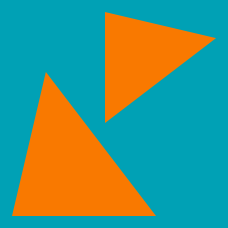Geometry

# Congruent and Similar Triangles: Level 3 Challenges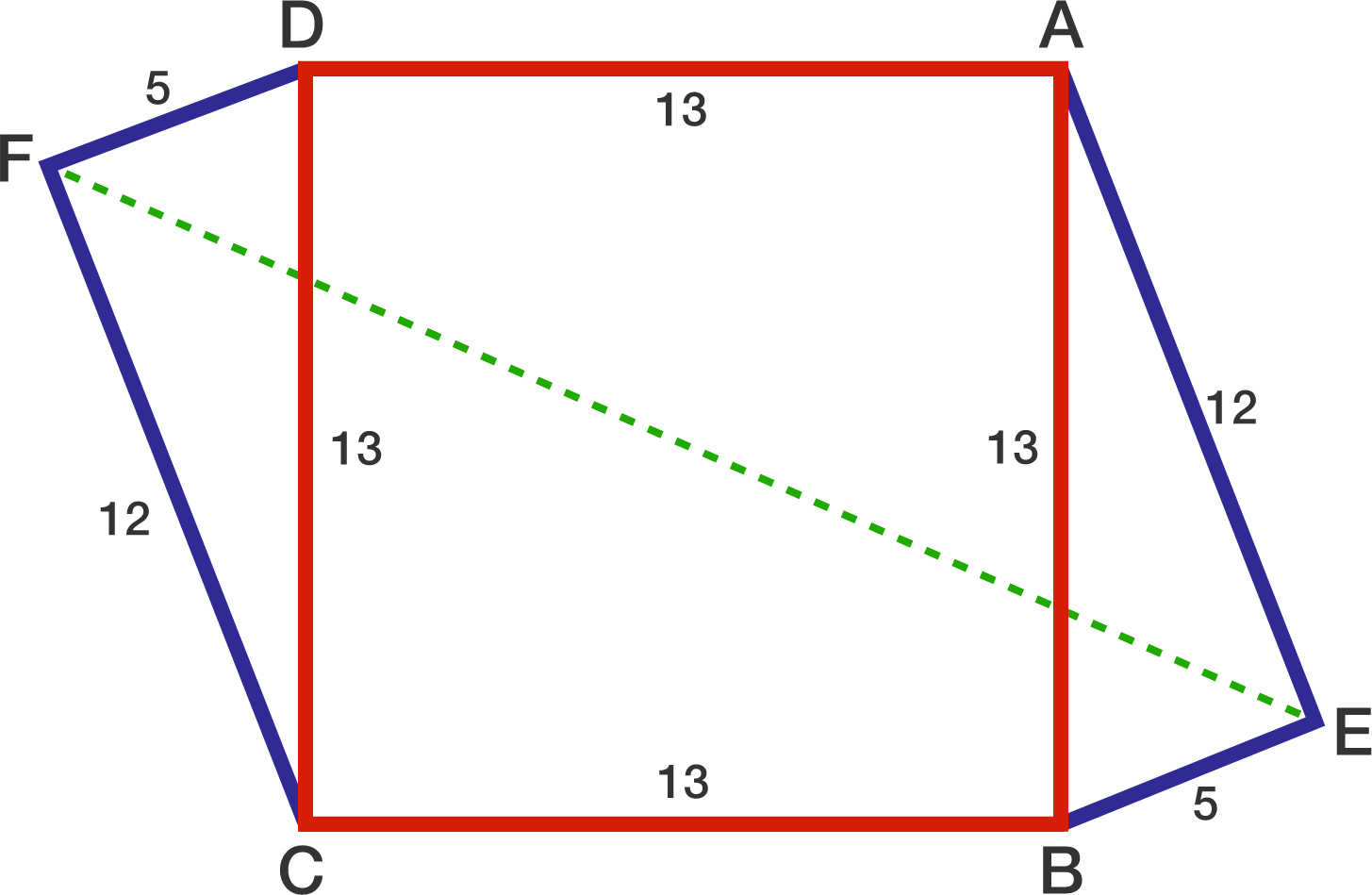$ABCD$ is a square with $AB=13$. Points $E$ and $F$ are exterior to $ABCD$ such that $BE=DF=5$ and $AE=CF=12$.

If the length of $EF$ can be represented as $a\sqrt b,$ where $a$ and $b$ are positive integers and $b$ is not divisible by the square of any prime, then find $ab$.

The area of the largest square which can be inscribed in a triangle with side lengths $3, 4, 5$ is $\dfrac{a}{b}$.

Find $a+b$, where $a$ and $b$ are coprime, positive integers.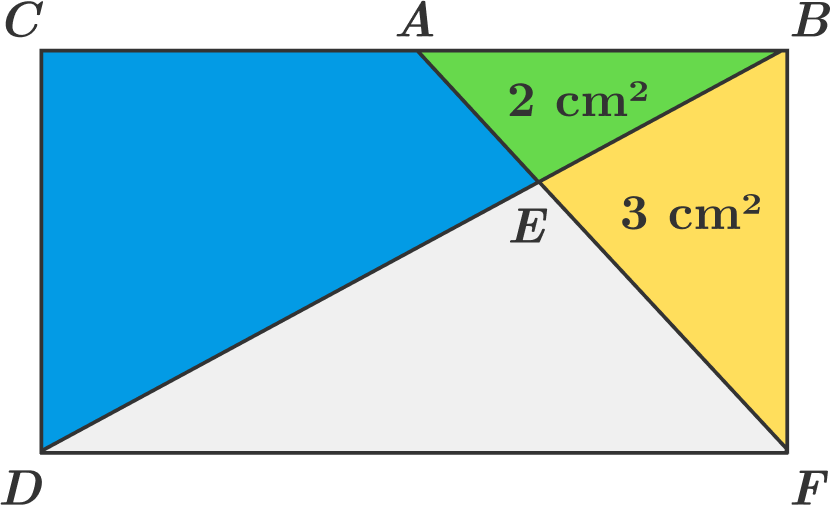$BCDF$ is a rectangle. Triangle $ABE$ has an area of $2 \text{ cm}^2$. Triangle $BEF$ has an area of $3 \text{ cm}^2$.

Find the area of the blue region (in $\text{cm} ^2$). Give your answer to 1 decimal place. (The figure is not drawn to scale. )

Note: This question is supposed to be harder than it looks like. Do not assume anything that is not stated in the problem! (Hint: Angle E is NOT 90 degrees, nor is A the midpoint of BC.)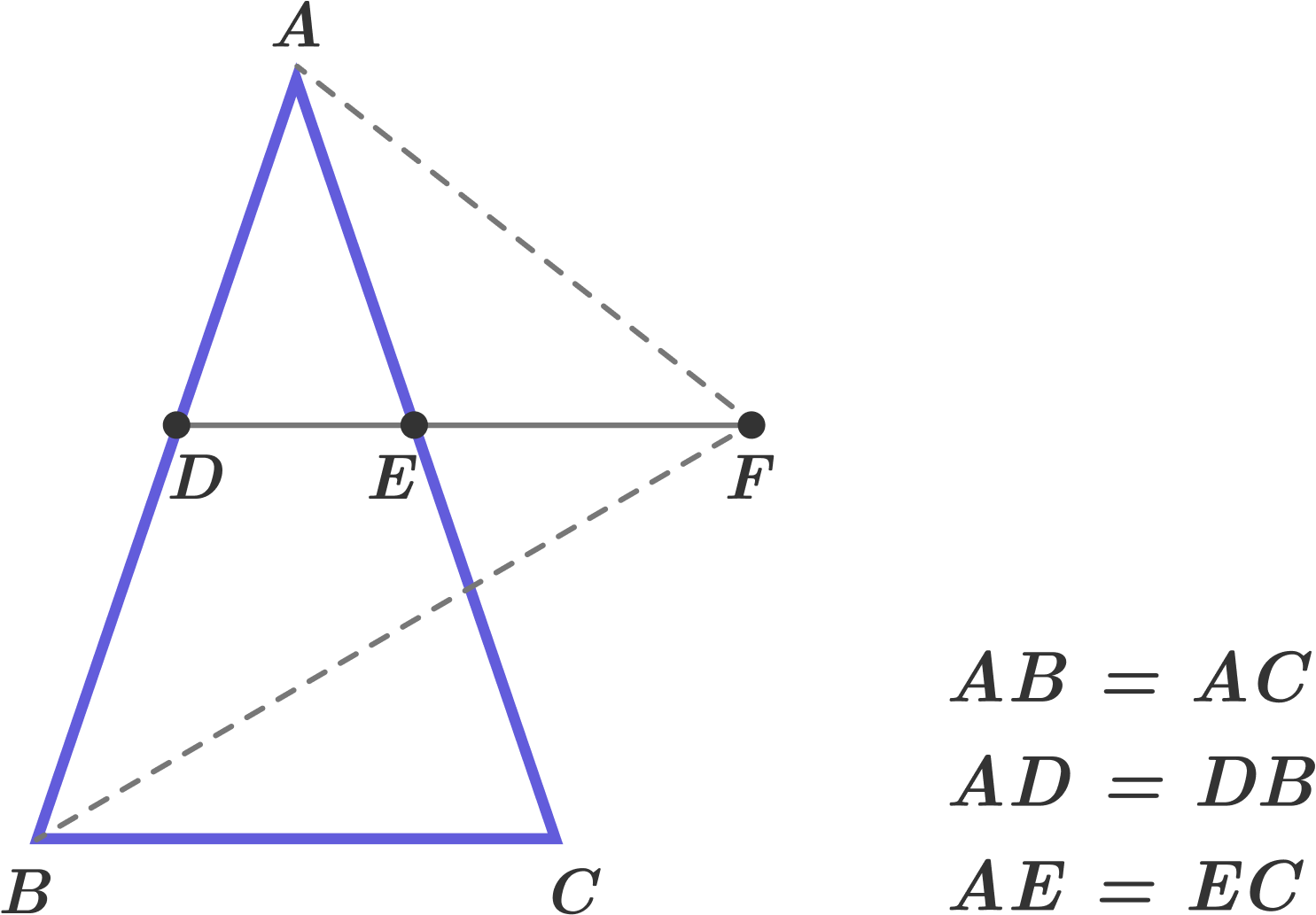If triangle $ABC$ is similar to triangle $BFA,$ then find $\dfrac{AB}{BC}.$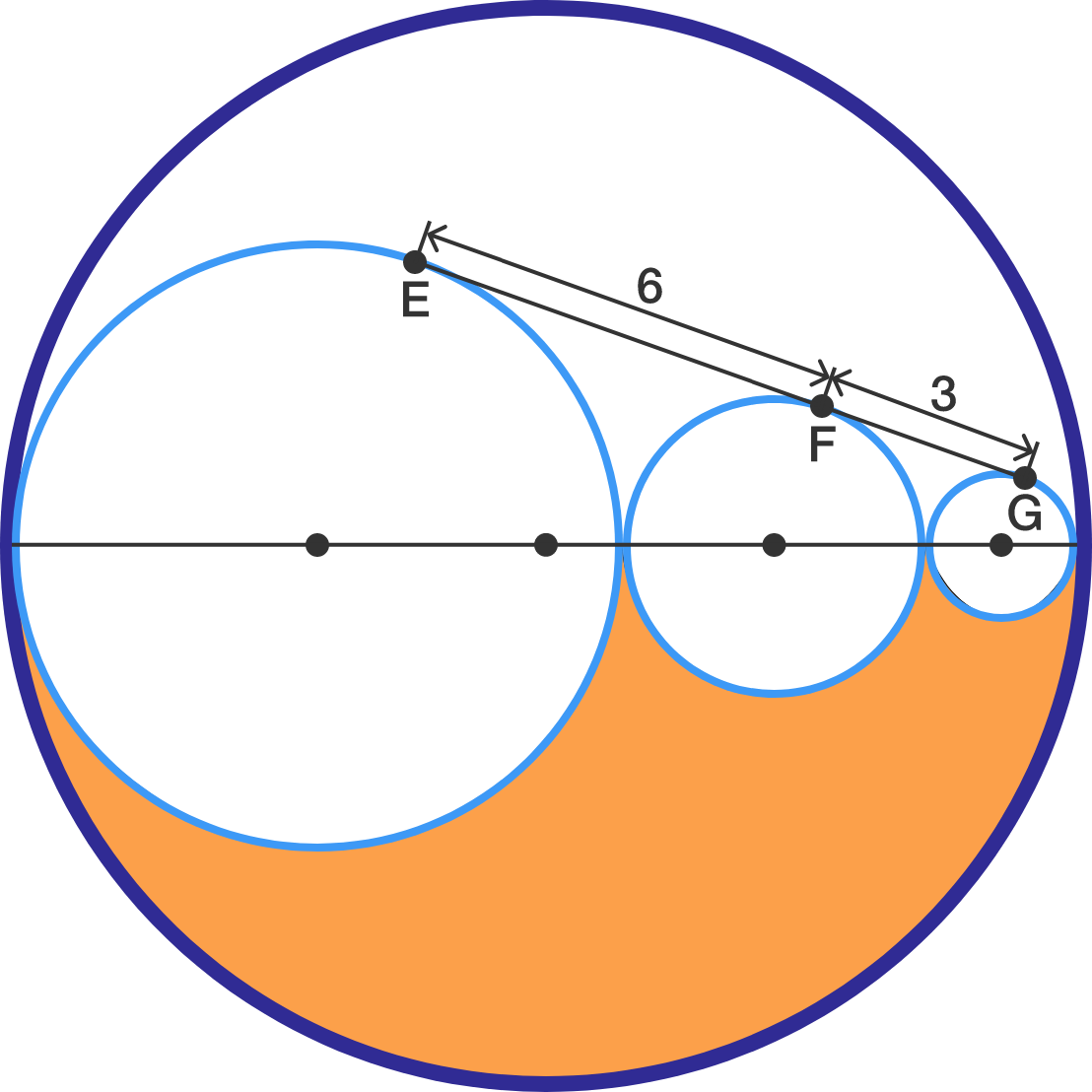There is a common tangent which intersects the three tangent circles shown above at $E,$ $F,$ and $G.$ If $EF = 6$ and $FG = 3$, find the area of the orange region. Use the approximation $\pi \approx \frac{22}{7}.$

×